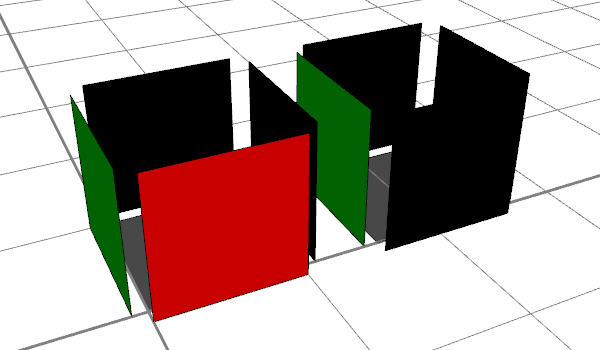# reverseNormals operation

### Syntax

• reverseNormals()

### Description

The reverseNormals operation reverses the face normals of the geometry of the current shape.

##### Note:

To check the orientation of the face normals, deactivate the "Use Two-Sided Lighting" option under Preferences/General/Grammar Core.

### Examples

##### Note:

Screenshots with deactivated "Two-Sided Lighting", thus the faces are black whose normals are facing away from the camera.

The generated geometry only is changed, not the scope. You can see this by the fact that the translation command in the example translates both the normal and inverted geometry in the same direction.

 Left box: face with index=0 not inverted ``````Lot --> extrude(world.up,1) comp(f) {side : Facade} Facade --> case comp.index = 0: color("#ff0000") # = red t(0,0,0.1) else: color("#00ff00") # = green t(0,0,0.1)`````` Right box: face with index=0 inverted ``````Lot --> extrude(world.up,1) comp(f) {side : Facade} Facade --> case comp.index = 0: color("#ff0000") # = red t(0,0,0.1) reverseNormals else: color("#00ff00") # = green t(0,0,0.1)``````### Advanced knowledge: Understanding the reversion process

Normals: The normal of a polygonal face is defined by the vertex order. By default, the order is counterclockwise.

Some advanced users may notice that after the reverseNormals command, the vertex order [black] is not only inverted (as in the classic mathematical definition of the normal inversion [blue]) but is offset by the value of 1 [red]. The reason for this is because the CityEngine often works with the "First Edge", which is defined by the vertex indices 0 and 1. Thus, in the reverseNormals command, the "First Edge" is retained.• black: standard vertex order of the face (counterclockwise)
• blue: classic reverted vertex order
• red: reverted vertex order as used in the CityEngine, with retained first edge.
##### Note:

Some game engines cannot display two-sided geometries, thus they display only those polygons whose normals are oriented towards the camera. Other polygons are not rendered. Therefore, it is particularly important to write cga code with this fact in mind when modeling for game engines.